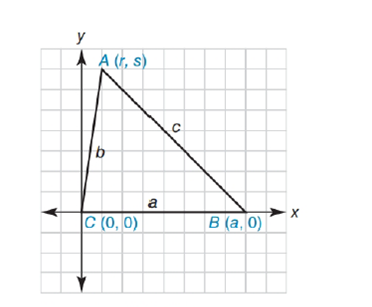Chapter 10.4, Problem 30E### Elementary Geometry for College St...

6th Edition
Daniel C. Alexander + 1 other
ISBN: 9781285195698

#### Solutions

Chapter
Section### Elementary Geometry for College St...

6th Edition
Daniel C. Alexander + 1 other
ISBN: 9781285195698
Textbook Problem
1 views

# Complete an analytic proof of the following theorem: In a triangle that has sides of lengths a ,   b , and c , if c 2 = a 2 + b 2 , then the triangle is a right triangle.To determine

To prove:

A triangle has sides of lengths a, b, and c, if c2=a2+b2, then the triangle is a right triangle

Explanation

Given figure is,

The above figure shows the triangle ABC.

And the coordinates of the quadrilateral ABC is as shown in the figure,

C0, 0, B(a,0) and A(r,s)

a is the length of CB

b is the length of AC

c is the length of AB

a=a-02+(0-0)2

a=a2

c=r-a2+(s-0)2

c=r-a2+s2

b=0-r2+(0-s)2

b=r2+s2

Substituting a, b, c values in the equation c2=a2+b2

### Still sussing out bartleby?

Check out a sample textbook solution.

See a sample solution

#### The Solution to Your Study Problems

Bartleby provides explanations to thousands of textbook problems written by our experts, many with advanced degrees!

Get Started

#### Find more solutions based on key concepts